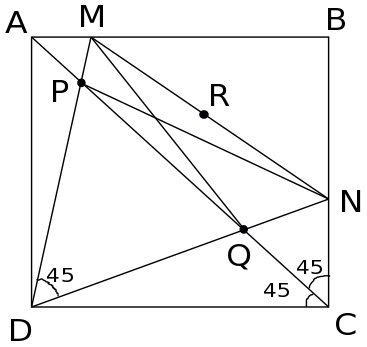# Problem #3

[RMO-1999]: Let ABCD be a square and $$M$$ & $$N$$ points on sides $$AB$$ & $$BC$$ respectively such that $$\angle MDN = 45^o$$. If $$R$$ is the mid point of $$MN$$, show that $$RP = RQ$$, where $$P$$ & $$Q$$ are the points of intersection of $$AC$$ with the lines $$MD$$ & $$ND$$

Solution:Join $$PN$$ and $$MQ$$. Since $$\angle PDN = \angle PCN$$, PDCN is a cyclic quadrilateral. It can now be inferred that $$\angle PND = \angle PCD = 45^o$$.

Now look at $$\triangle PDN. Since \angle PDN = \angle PND = 45^o, => \angle NPD = \angle NPM = 90^o$$. Similarly, $$\angle MQD = \angle MQN = 90^o$$.

Since $$\angle NPM = \angle MQN = 90^o$$, $$MPQN$$ is also a cyclic quadrilateral. Furthermore, $$MN$$ becomes the diameter of the circumcircle of $$MPQN$$ since $$MN$$ project $$90^o$$ on the sector. Since $$R$$ is the midpoint of $$MN, => R$$ is the centre of the circumcircle of $$MPQN$$. Thus, $$RP = RQ$$